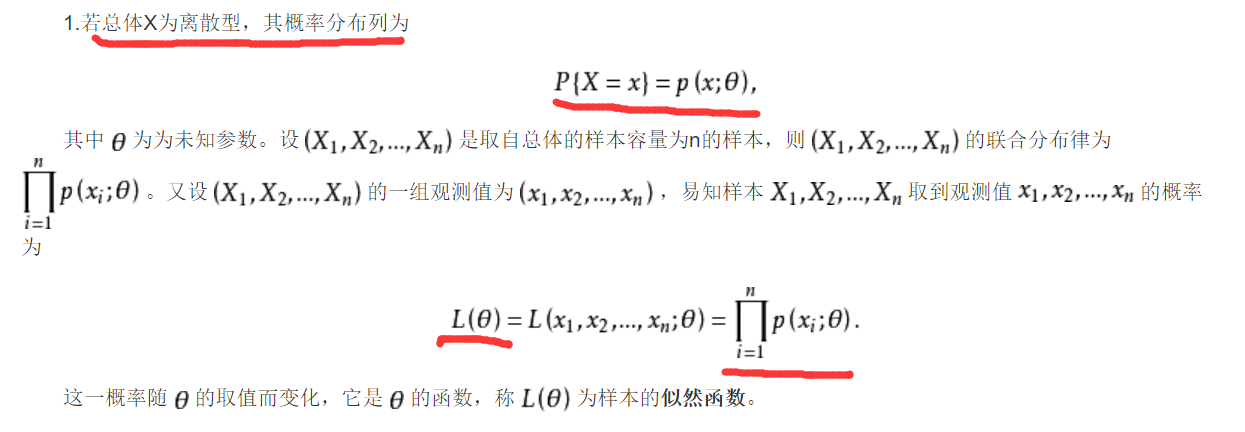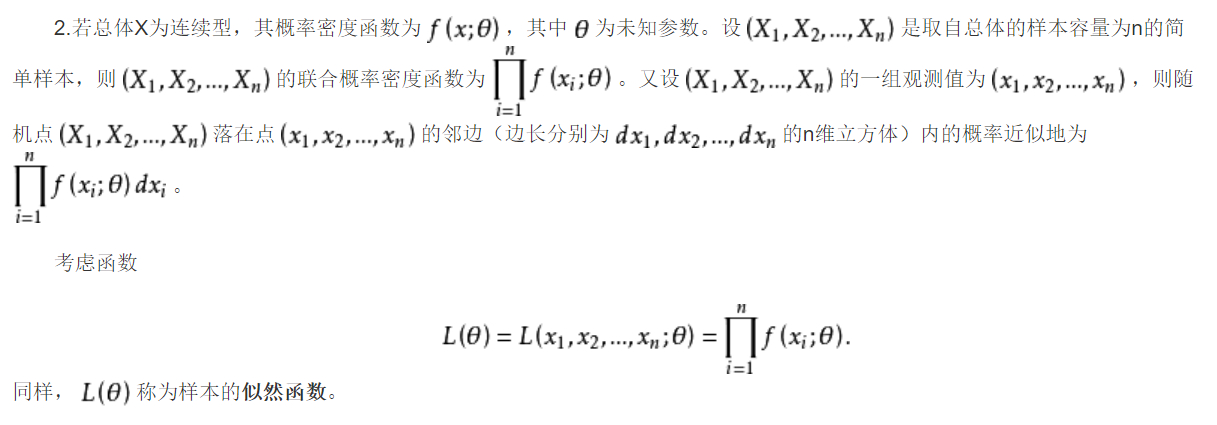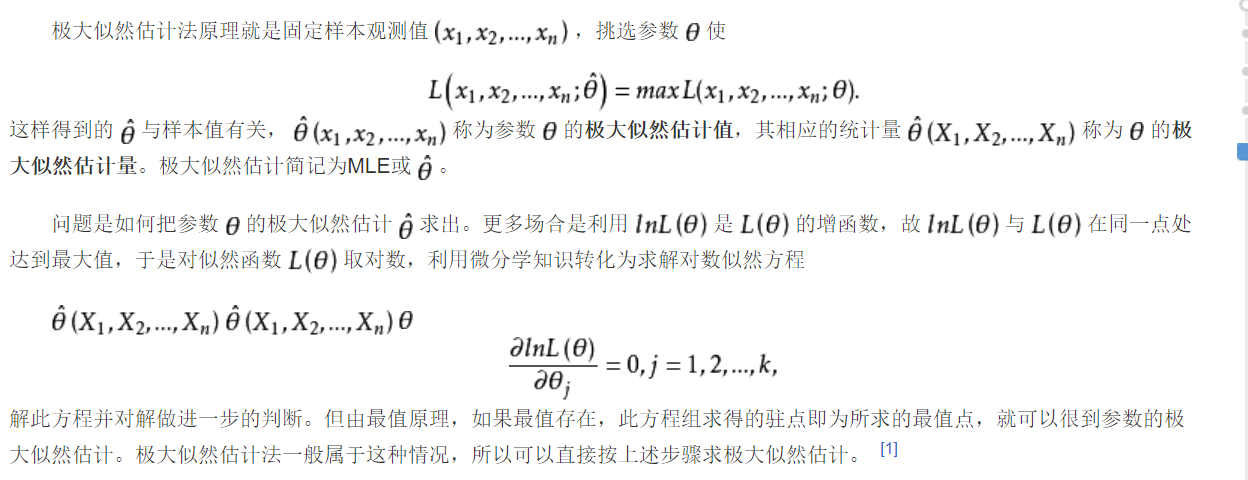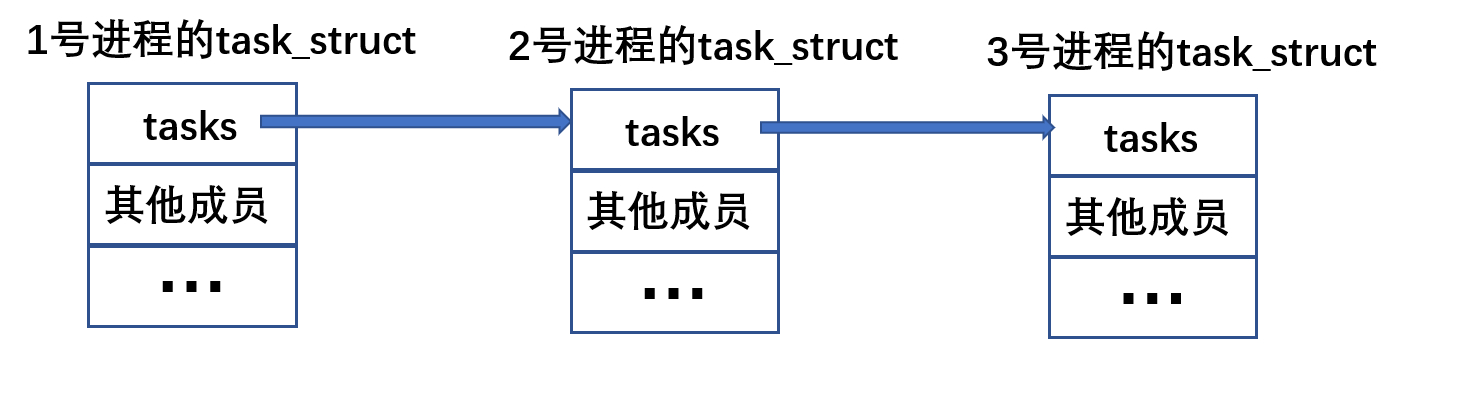Doragd 坚持思考，坚持输出。 2019-05-17T10:25:19.061Z http://blog.doragd.cn/ Doragd Hexo 参数估计 http://blog.doragd.cn/2019/04/20/ML-Parameter-Estimation-002/ 2019-04-20T08:25:20.000Z 2019-05-17T10:25:19.061Z
• 总体分布的表示：以$f(x;\theta_1,…,\theta_k)$ 记其为概率密度函数（连续型）或其概率分布函数（离散型）为总体分布。这个分布具有$k$个未知参数$\theta_1,…,\theta_k$ 。例如正态分布总体$N(\mu,\sigma^2)$ 有$\theta_1=\mu$,$\theta_2=\sigma^2$。例如二项分布，只有一个参数，就用$\theta$ 表示。

• 参数估计的点估计：

• 设有了从总体中抽出的样本$X_1,…,X_n$ (独立同分布)，要依据这些样本对参数$\theta_1 ,\theta_2,…,\theta_k$ 的未知值进行估计，或估计它们中的一部分，或它们的的某个已知函数$g(\theta_1,\theta_2,…)$ 。
• 例如要估计$\theta_1$
• 我们要构造适当的统计量$\hat{\theta_1}=\hat{\theta_1}(X_1,X_2,…,X_n)$ 。
• 每次有了样本，就带入上面的函数算出一个值，作为$\theta_1$ 的估计值
• 这种统计量叫做xxx的估计量。
• 由于未知参数$\theta_1$是数轴一个点，我们要用一个点去估计另一个点，叫做点估计。
• 点估计的方法有几种，同一个参数往往可以用若干看起来合理的方法去估计，因此要有一种判断优劣的准则。
• 矩估计

• 极大似然估计

• 设总体有分布$f(x;\theta_1,\theta_2,…,\theta_k)$ ， 从总体中抽出的样本$X_1,…,X_n$ (以后默认都是独立同分布)，则样本的联合分布为(即$(X_1,X_2,..,X_n)$的分布)：注意！单个样本的分布和总体相同，联合分布不同！
• 用概率密度函数表示为
• $f(x_1,x_2,…,x_n)=f(x_1;\theta_{1:k})f(x_2;\theta_{1:k})…f(x_n;\theta_{1:k})$
• 因为独立！！所以可以直接拆开乘
• 记为$L(x_1,…,x_n;\theta_1,…,\theta_k)$
• 固定$\theta$ ,若有$L(Y_{1:n})>L(X_{1:n})$ 则说明观察时出现$Y_{1:n}$ 点的可能性更大
• 固定$X$ ,若有$L(\theta’)>L(\theta’’)$ 则说明被估计的参数$\theta_{1:k}$ 是$\theta’$ 的可能性更大
• 固定$X$ 时，$L(\theta)$ 称为似然函数

• 反映了在观察结果已知的情况下，$(\theta_1,…,\theta_k)$ 的各种值的似然程度

• 这里有点像贝叶斯公式的推理：由结果推各种原因的概率。
• 这里参数$\theta_{1:k}$ 有一定的值（虽然未知），并非事件或随机变量，于是用“似然”这个词
• 自然！我们应该用似然程度最大的那个点$(\theta_{1:k}^)$ ,即满足下列条件的那个$(\theta_{1:k}^)$作为参数的估计值

$L(X_1,…,X_n;\theta_1^,…,\theta_k^)=\max_\limits{\theta_1,…\theta_k}L(X_1,…,X_n;\theta_1,…,\theta_k)$

• 因为在已得样本的条件下，这个看起来最像是真的参数值！！！（这个看起来像即似然！最像即似然程度最大）。

• 这个估计$(\theta_{1:k}^*)$ 就叫做$(\theta_{1:k})$ 的极大似然估计。

• 为什么要最大化这个$L$ 其实有更好的解释：以下摘自CSDN和百度百科

https://blog.csdn.net/baidu_38172402/article/details/89074482

对于这个函数：$f(x;\theta)$
输入有两个：$x$表示某一个具体的数据；$\theta$表示模型的参数。

如果$x$未知，$\theta$ 已知，则称为概率函数，表示给定模型，不同样本点出现的概率是多少

如果$x$已知，$\theta$ 未知，一般写作$L(\theta|x)$ 。称为似然函数。表示对于不同模型参数下，出现$x$这个样本点的概率是多少

我们当然希望极大化这个函数！！使得出现$x$这个样本点的概率最大呀！！

可是，上面的是$L$ 似乎不是$P$ ，即不是概率，而是概率密度函数？最大化L等价于最大化P吗？

是的！！！我们看百度百科！！对于离散型，用分布列进行描述。可以看到两者自然等价。

而对于连续型注意：上面还提到了样本和样本的观测值！！！学习一下！

反正，虽然还是对那个$n$维立方体看不太懂，但是可以看到最大化$L$确实是和最大化$P$ 等价的

后面的步骤，百度百科讲的很好了！如何求极大似然估计。我觉得用语也比那本书更规范。比如提到了样本和样本观测值，极大似然估计值和极大似然估计量。注意：如果$f$ 对参数不可导，或者$f$根本不连续，此时则似然方程就不能用，必须要回到max那个式子去求。还要注意求出来的点可能有几组，是极值点，要验证去找最值点。

注意：一般说来，它相对其他估计方法，比较优良，当然也有不好的情况。与矩估计不同，极大似然估计要求分布需要有参数的形式。如果对总体分布毫无所知，而要去估计其期望，方差时，这种方法无能为力！！

• 贝叶斯估计

• 对于贝叶斯学派，假设抽样前，已经对$\theta$ 有一定的知识，叫先验知识，进一步的，学派要求这种知识要用某个概率分布表示出来，这个分布就叫先验分布(先验概率$P(\theta)$)

• 如何获得这种分布（或者先验概率$P(\theta)$）：
• 学派必须要求我们通过历史经验，甚至主观认识去假定一个这样的分布出来！（即这里可以使用主观概率）
• 获得了分布，如何得出参数的估计？
• 首先，通过条件概率公式，获得$\theta$ 的条件概率
$P(\theta|X_1,…,X_n)=\frac{P(\theta)P(X_1…X_n|\theta)}{P(X_1,…,X_n)}=\frac{P(\theta)P(X_1|\theta)…P(X_2|\theta)}{P(X_1,…,X_n)}$

• $P(X_1,…,X_n)$ 是边缘概率，通过$(\theta;X)$ 的联合概率分布推导出。

• $P(X_1,…,X_n)=\sum_{\theta}{P(\theta;X)}$

• $P(\theta|X_1,…,X_n)$ 代表了我们对参数的认识，综合了参数的先验知识和样本带来的信息，被称为后验概率，因为是在做了试验之后取得的。

• 贝叶斯学派下一个重要观点：在得出后验分布后，对参数$\theta$ 的任何统计推断都必须基于这个分布。

• 具体如何使用。有不同的准则和自由度。一个常用的方法是取后验分布式子的均值作为参数的估计！

• 而极大后验估计，就是

• $\hat{\theta}_{MAP}=arg\max{P(\theta|X)}$

• 即：$arg\max{P(\theta)P(X|\theta)}$

即：$arg \max{\log{P(\theta)}+\log{P(X|\theta)}}$

即：$arg \min (-\log{P(\theta)}-\log{P(X|\theta)})$

• 找参数，使得后验概率最大

• 这他妈不就是负对数似然加一个先验项吗！！！！！

• 垃圾CSDN!!!

• 似然函数：$L(\theta|x)=P(x|\theta)\equiv P(x;\theta)$

]]>
<ul> <li><p>总体分布的表示：以$f(x;\theta_1,…,\theta_k)$ 记其为概率密度函数（连续型）或其概率分布函数（离散型）为总体分布。这个分布具有$k$个未知参数$\theta_1,…,\theta_k$ 。例如正态分布总体$N(\mu,\sigma^ 总体和样本 http://blog.doragd.cn/2019/04/20/ML-Parameter-Estimation-001/ 2019-04-20T07:40:27.000Z 2019-04-20T09:18:03.405Z • 当我们用试验或者观察的方法研究一个问题，首先要通过试验取得必要的数据，然后对数据进行分析，以便对所提问题做出尽可能正确的结论 • 尽可能正确：数据带有随机性的误差，这种误差除了测量不准带来的误差以外，关键的是我们所获得的数据仅仅是所研究事物的一小部分，究竟是哪一部分则是随机的，所以分析得出的结论一般是有错误的。 • 不过我们想错误愈小愈好。需要用到数理统计的方法 • 数理统计：用概率论和数学方法，研究怎么收集带有随机误差的数据，在设定的统计模型之下，对数据进行统计分析，以对所研究的问题进行统计推断。 • 例子：研究元件的平均寿命。（书上第二个问题是关于假设检验的，先跳过） • 我们事先假定元件寿命服从指数分布。（统计模型） • 如果我们知道了分布中参数$\lambda$的值，则我们可以直接推出平均寿命为$1/\lambda$• 但实际上这个参数往往是未知的。我们只好从大批元件中在同等条件下随机抽出若干个，测出其寿命为$X_1,…,X_n$（收集的数据） • 一个自然的想法是用$n$个元件寿命的均值去估计$\lambda$。（统计分析和推断） • 这种问题叫做！！！参数估计！！！！非常重要的一个问题！！ • 总体 • 所研究问题的对象的全体构成的集合。总体随研究范围而定，比如中国的大学，杭州的大学。 • 我们研究的总体中的个体，实际上只关心一些指标，比如要研究大学生的身高，那么我们就关注大学生的身高这一指标值。那么总体就是这些身高值组成的集合。 • 总体单单有这样一堆杂乱无章的数还不行，没有什么数学或概率上的性质。比如元件的寿命是一堆数字，大学生身高也是一堆数字。 • 所以！！我们要研究的是赋有一定概率分布的总体，称为统计总体 • 总体分布不同，分析方法不同。 • 比如元件寿命分布为指数分布，大学生身高分布为正态分布 • 即使两个总体内个体性质根本不同，但只要有同一的概率分布，则可以视为同一类总体 • 比如人的寿命服从指数分布，元件啊的寿命也服从指数分布。 • 更准确地说：总体分布是一个概率分布族的一员。 • 因为我们假定元件寿命服从指数分布，并没有指定$\lambda$取值 • 比如元件寿命是一个指数分布族中的一员。(包含一个参数$\lambda$，叫做单参数分布族) • 正态分布族是双参数分布族 • 另外，有时我们只是假设总体具有一定概率分布，并不知道其数学形式，这种总体叫非参数总体 • 还有就是实际上总体中个体数总是有限的，有限总体的相应分布是离散的，其形式与个体总数有关，且缺少一个简洁的数学形式。我们可以用一个连续分布去逼近离散分布。当总体所含个数特别多时，这种逼近所带来的误差可以忽略不计。尤其是正态分布，对许多实际问题的总体分布都给了足够好的近似！背后的原因和大数定理有关！ • 注意：一个物体的真实重量未知，我们多次量测去估计它。该试验的总体不是这个物体！而是所有可能的量测结果组成的集合！！！我们只需要说量测结果是随机的，服从某某分布即可。 • 样本 • 从总体中在抽出的一部分个体。（注意：每个个体抽出机会要等同） • 我们兴趣不在个体本身，而是在个体的某一特征值（比如身高）， • 描述为：$n$个样本，$X_1,…,X_n$。尽管他们有具体的值，但实际上也是随机变量，因为抽到哪一个个体是随机的。所以样本$X_1,…,X_ n$,某一次的观测值我们记为$x_1,…,x_n$• 当总体个数足够多，抽出$n$个样本不会改变其原有分布，因此，这些样本是独立同分布的，其分布即为总体的分布。（我们主要研究这种情形，即总体个数足够多的情况） • 统计量 • 完全由样本所决定的量叫做统计量 • 比如$\bar{X}=(X_1+X_2+…+X_n)/N$就是一个统计量，所谓“完全”指统计量只依赖于样本，里面不能有其他未知的量。尤其是，它不能依赖总体分布中所包含的参数。比如$(X-\mu)$不是统计量。 • 统计量可以看作是对样本的一种加工，将样本所含某一方面的信息集中起来。比如$\bar{X}$可用于估计$\mu$。而如果想了解总体方差$\sigma^2$，则需要用$S^2=\sum_\limits{i=1}^n(X_i-\bar{X}^2)/(n-1)$称作样本方差 • 样本矩（先略掉 • ]]> <ul> <li>当我们用试验或者观察的方法研究一个问题，首先要通过试验取得必要的数据，然后对数据进行分析，以便对所提问题做出尽可能正确的结论<ul> <li>尽可能正确：数据带有随机性的误差，这种误差除了测量不准带来的误差以外，关键的是我们所获得的数据仅仅是所研究事物的一小部分 随机变量和概率分布 http://blog.doragd.cn/2019/04/20/ML-Probability-and-Statistics-002/ 2019-04-20T02:52:03.000Z 2019-04-20T07:21:25.494Z • 随机变量是其值随机会而定的变量，一个随机试验有许多可能的结果，到底出现哪一个要看机会，有一定的概率，在试验前，我们不能预知它取何值，试验后，取值就定了 • 实际上就是用随机变量来表示一个随机试验可能出现的结果 • 比如：在某厂大批产品中随机抽出100个，其中所含废品数$X​$• 一个月内某交通路口的事故数$X$• 考虑一个打靶的试验，其命中位置由坐标($X,Y$)刻画,$X,Y$都是随机变量。而$(X,Y)$称为一个二维随机向量或者二维随机变量，多维随机向量$(X_1,X_2,…X_n)$的意义据此推广 • 离散型随机变量：取有限或无限个值，但可以一个一个毫无遗漏，逐一排列出来 • 在某厂大批产品中随机抽出100个，其中所含废品数$X
• 连续型随机变量：可能取值无穷多，且不能一个一个毫无遗漏，逐一排列出来

• 比如称重一个物体重量的误差，如果没有指定范围，则取值就是$(-\infty,+\infty)$
• 电视机的寿命$(0,+\infty)$
• 连续是一个数学上的抽象，实际上任何量都有一定单位，只能在该单位下量到一定程度，故必然是离散的，当单位极小时，在某一范围内可能会非常密集，不如视为连续量更好处理
• 概率分布

• 研究一个随机变量，不仅仅是看它能取哪些值，更要看取各种值的概率
• 概率分布是指概率1在可能值集合$\{a_1,a_2,…\}$上的分布情况，称作随机变量$X$的概率分布
• 也就是说概率分布描述了随机变量取各种值的概率
• 有许多种表示概率分布的形式。比如分布律，概率分布函数，概率密度函数。不同类型变量采用不同的描述概率分布的形式，主要是怎么方便怎么来！关键是这些描述的都是概率分布！
• 离散型随机变量：用分布律来表示，更方便的是概率分布函数$F(x)=P(X\le x)$

• 二项分布$B(n,p)$
• 泊松分布$P(\lambda)$
• 超几何分布
• 负二项分布
• 连续型随机变量：变量的取值充满一个区间，无法一一排出，若指定一个数$a$ 则变量$X$恰好是$a$ 丝毫不差是不可能的，

• 例在靶子上指定一个点，射击时恰好命中该点的概率是0！！

• 刻画连续型随机变量概率分布最好用的就是概率密度函数

• 概率密度函数$f(x)=F’(x)$
• 概率密度函数可以用面积描述x落在某个区间的概率，而概率分布函数则不容易直观看出
• 正态分布

• 首先！正态分布是一种分布，刻画随机变量$X$取各种可能值的概率，也可以说是概率1在可能值集合上的分布情况。
• 正态分布常用概率密度函数来描述！！
• 如果说一个随机变量具有如下概率密度函数,那么我们就说这个$X$具有正态分布，或者说服从正态分布，记为$X\sim{N(\mu,\sigma^2)}$
• 指数分布

• 威布尔分布

• 均匀分布

• 多维随机变量(随机向量)

• 设$X=(X_1,X_2,…,X_n)$ 为一个$n$维向量，其每个分量是一个一维随机变量，则$X$是一个$n$维随机变量(随机向量)
• 多维离散型随机变量的分布
• 多维离散型随机变量：每个分量是一维离散型随机变量
• 多维离散型随机变量的概率分布：可以把所有情况列举出来即可
• $P(X_1=2,X2=1)=1/3$, $P(X_1=2,X_2=2.5)=1/4$,$P(X_1=5,X_2=3)=5/12$
• 上面这个就叫概率分布！！不用拘泥于形式！因为概率分布就是在描述随机变量取不同可能值的概率
• 多项分布
• 连续型随机向量的分布
• 设$X=(X_1,X_2,…,X_n)$ 是一个$n$维随机向量，其取值可视为$n$维欧式空间$\mathbb{R}^n$ 中的一个点，如果X的全部取值能充满$\mathbb{R}^n$ 中的一个区域，则称它是连续型的。
• 描述多维随机向量的概率分布，最方便是的概率密度函数，分布函数极少用
• 二维正态分布
• 几点事项：
• 连续型的随机变量不能简单定义为分量是连续型，见书吧。先跳过
• 边缘分布 和联合分布
• 设$X=(X_1,…,X_n)​$ 为一个$n​$维随机向量，$X​$有一定的分布$F​$
• 这个$F$指$X$在取各种情况时的概率
• 这个$F$也被称作$X_1,…,X_n$ 的联合分布
• $X$的每个分量$X_i$ 都是一维随机变量，故都具有各自的分布$F_i$
• 这个$F_i$ 称作$X$或其分布$F$的边缘分布
• 比如离散型变量的联合分布：$P(X_1=a,X_2=b)$，可以简记作$P(a,b)$
• 其边缘分布为$P(X_1=a)$ 和$P(X_2=b)$
• 一般说来：$\forall x\in X, P(X=x)=\sum_y P(X=x, Y=y)$
• 连续型联合分布与边缘分布：概率密度函数$f(x_1,x_2)$ ，其边缘密度函数为$f_1(x_1)$ ，$f_2(x_2)$
• 一般说来：$f_\mathbb{x}(x) = \int f(x,y)dy$
• 注意：联合分布可以导出任一分量的(边缘)分布。但是即使知道了所有分量的分布，则不能决定联合分布。因为边缘分布只考虑单一分量的情况，未涉及分量之间的关系，这个信息是包含在联合分布之内的。
• 条件概率分布

• 一个随机向量或者变量的条件概率分布就是，在某种给定条件之下的$X$的概率分布
• 条件概率分布可以帮我们去研究某个变量对另一个变量的影响，研究变量间的相依关系
• 条件概率公式可以导出：(X,Y)的联合分布与条件概率分布的关系：$P(X=a,Y=b)=P(Y=b|X=a)P(X=a)$
• 注意, 必须满足 $P(X=a)>0$, 否则对于永远不会发生的事情讨论条件概率无意义.
• 基于条件概率, 任意多维随机变量的联合分布都可以写成其中任意一个随机变量的条件概率相乘的形式
• 比如考虑一个三元分布

• $P(a,b,c)=P(a|b,c)P(b,c)=P(a|b,c)P(b|c)P(c)$

• 反正就一步步把这个多元分布分解就好！！！！！

• $P(c):c$ 发生的概率, 通常已知.
$P(b|c):c$ 发生的条件下, 观察到 $b$ 的概率, 通常从数据中挖出.

$P(a|b,c):b,c$同时发生的条件下, 观察到 $a$ 的概率, 通常从数据中挖出.

• 条件独立

• 两个随机变量 $X,Y$ 相互独立的数学描述：$P(X, Y)=P(X) P(Y)$ 。记作：$X \perp Y$ 。

• 两个随机变量 $X,Y$关于随机变量 $Z$条件独立的数学描述：$P(X, Y | Z)=P(X | Z) P(Y | Z)$ 。

记作：$X \perp Y | Z$ 。

• ]]>
<ul> <li><p>随机变量是其值随机会而定的变量，一个随机试验有许多可能的结果，到底出现哪一个要看机会，有一定的概率，在试验前，我们不能预知它取何值，试验后，取值就定了</p> <ul> <li>实际上就是用随机变量来表示一个随机试验可能出现的结果</li> <li>比如：
事件的概率 http://blog.doragd.cn/2019/04/19/ML-Probability-and-Statistics-001/ 2019-04-19T14:37:03.000Z 2019-04-20T02:51:25.684Z
• 概率，又称或然率，几率，是表示某种情况(事件)出现可能性大小的一种数量指标，介于01之间
• 事件是对某(种)些情况的“陈述”
• 它是否发生，要到有关的“试验”有了结果才知道
• 这里的试验，对人而言，只是一个观察者，记录试验结果，试验的全部可能结果在试验前就明确的，当然我们可能不能确切知道一个试验的全部结果，但可以知道它不会超过某个范围，可以用这个范围来表示试验的全部可能结果，甚至取$(-\infty,+\infty)$ 也可以。尽管我们明知一些结果是不会出现。这样的抽象有很大的好处。
• 由此，我们可以定义所谓“陈述”是什么，即事件是什么
• 事件是试验的全部可能结果中的一个确定的部分。
• 例子：
• 试验：观察明天下午6时的天气状况(假设以降雨量$x$为衡量)
• 试验的全部可能结果：$x\in[0,+\infty)$
• 事件：$A=\{明天下午下雨100mm\}$
• 例子：
• 试验：抛一枚硬币，观察掷出的点数结果
• 试验的全部可能结果：掷出的点数为$\{1,2,3,4,5,6\}$
• 事件：$A=\{掷出偶数点\}=(2,4,6)$
• 如果做一次实验，丢出2点，则我们说”事件A发生了”
• 在概率论上，把单一的实验结果叫做一个基本事件，也常称事件为“随机事件”或“偶然事件”。
• 随机的意思是说，事件是否在某次试验中发生，取决于机遇
• 随机事件的极端情况：必然事件和不可能事件，其概率为1和0
• 等可能：从一个试验的条件和实施方法上看，我们找不到任一可能结果比其他可能结果更容易发生，只好认为所有可能结果在试验中有同等可能的出现机会，称这样的试验结果是”等可能的“
• 古典概率：设一个试验有$N$个等可能的结果，事件$E$包含其中$M$个，则事件$E$的概率为$P(E)=M/N$.
• 古典概率是客观的，因为等可能的结果是基于客观事实(比如骰子是绝对均匀且严格正六面体)，不是主观设想的
• 古典概率的计算基于排列组合。
• 古典概率的局限性:只能用于全部试验结果为有限个，且等可能性成立的情况
• 几何概率：此时试验结果是无限多个的，等可能性也引申到了等面积，体积，长度等
• 概率的统计定义：
• 一个事件出现的可能性大小(概率)，用在同等条件下的大量重复试验中出现的频繁程度(频率)来刻画。
• 频率是事件概率的估计，更精确地说概率就是试验次数无限增大时频率的极限
• 这个定义的重要性在于
• 提供了一种估计概率的方法
• 提供一种检验理论或假设正确与否的准则：假设有一定的理论和假设，算出事件的概率，可以通过多次重复试验来观察事件的频率，如果与算出的概率相去甚远，则理论可能有误
• 概率的公理化定义：给出了一些概率所具有的一般性质
• 古典概率计算
• 事件的关系：同一试验下的两事件$A,B$
• $B$包含$A$：
• 从事件发生观点：事件A发生时，B必发生
• 从试验结果观点：A的试验结果一定包含在B中
• 注意：只要事件A的一个试验结果发生，则事件A发生
• 比如：A是丢到偶数点，结果是2，则A发生。而B如果定义为丢到的点数大于2。则B包含A！
• B=A：两个事件相互包含
• 若A发生，B一定发生。反之也成立时。
• A,B互斥：
• 试验结果无交集
• 两事件至多发生一个
• A，B对立
• 两事件不同时发生，但一方不发生，另一方一定发生
• 事件的和，并：A,B至少发生一个
• 注意定义事件$C=\{A+B\}=\{A,B至少发生一个\}​$ 只是一种陈述，不是说A,B肯定至少发生一个。必须要看试验结果。如果实验结果是A发生，则说“事件C发生了”。
• 事件的积，交：A,B同时发生
• $C=\{A\cdot{B}\}=\{A,B同时发生\}$
• 事件的差：A发生，B不发生
• $C=\{A-B\}=\{A发生，B不发生\}$
• 概率的加法公理：若干两两互斥事件之和的概率等于各事件概率之和

• $P(A_1+A_2+…)=P(A_1)+P(A_2)+…​$
• 条件概率：
• 条件概率就是附加一定条件下计算的概率
• 这个条件不是指决定实验的那些基础条件(比如正六面体)，而是另外附加的条件
• 所谓的条件的形式可以归结为：已知某某事件发生的情况下！！！
• 在给定B发生的条件下，A的条件概率为：$P(A|B)=P(AB)/P(B)$
• 即AB同时发生的概率除以B发生的概率
• 计算时，可以根据公式来，也可以根据实际意义来
• 事件的独立：若$P(A)=P(A|B)$ ，即B的发生与否对A的发生毫无影响，则称A,B独立
• 此时有：$P(AB)=P(A)P(B)$ 概率的乘法定理！
• 两事件满足上式与两事件独立等价
• 我们实际上不用上式判断是否等价，而是根据事件的实际意义去分析两者是否有关联，从而判断是否独立，从而可以应用上式
• 比如：两个机床的生成是彼此不相干的，所以各自生产的废品数的这类事件是独立的
• 但是有时未经周到的直观也会造成问题
• 例子: $B=\{至少一个骰子掷出1\}$，$A=\{三个骰子掷出点数至少有两个一样\}$
• 初看两者独立。但是考虑$P(A|\bar{B})$ 是从5个数取3个数 ，两个点一样的概率。$P(A)$ 是从6个数取3个数，两个点一样的概率。明显有$P(A|\bar{B})>P(A)$ ，则有$P(A)>P(A|B)$
• 多个事件独立

• 对一系列事件，任意取出多个事件满足$P(A_1A_2…A_m)=P(A_1)P(A_2)…P(A_m)​$ 则这一系列事件相互独立
• 同时，多个独立的事件的之积的概率=各事件概率的乘积
• 独立事件的任一部分也独立，独立事件决定的事件也独立
• $A,B,C,D$ 相互独立，则$A,C$ 或$B,C,D$都是相互独立的
• $A,B,C,D$ 相互独立，则$A-C$与$B+D$ 也独立
• 一系列事件相互独立，将里面任一部分改成对立事件时，所得事件也相互独立
• 一些事件如果任意两个都独立，则它们两两独立
• 相互独立，则一定两两独立(因为一个是取任意多，一个是取两个)
• 两两独立，不一定相互独立
• 全概率公式

• 设$B_1,B_2,…$为有限个或无限个事件，他们任意两个都互斥（$B_iB_j=\empty$），且在每次试验中至少发生一个($B_1+B_2+…=\Omega$)。这一组事件叫做完备事件群。注意任一事件与其对立事件组成一个完备事件群
• 考虑任一事件$A​$ ,因$\Omega​$ 为必然事件，则$A=A\Omega=AB_1+AB_2+…​$ ，且$AB_1,AB_2,…​$两两互斥
• 根据加法定理有：$P(A)=P(AB_1)+P(AB_2)+…$
• 根据条件概率公式：$P(AB_i)=P(B)P(A|B_i)$
• 有全概率公式：$P(A)=P(B_1)P(A|B_1)+P(B_2)P(A|B_2)+…$
• 在复杂情况下，计算$P(A)$ 不行，但是$A$总是随着某个$B_i$ 伴出，可以构造这样的一组$B_i$ 简化计算
• 可以把$B_i$ 看作导致$A$发生的一种可能途径，不同的$B_i$ 发生的概率$P(B_i)$ 视作权重，则$P(A)$ 是所有的$P(A|B_i)$ 的加权平均
• 贝叶斯公式

• 在全概率公式的假定下，有

• 从形式上看，这个公式只是条件概率定义和全概率公式的简单推论，但其富含哲理和现实意义

• 如果我们把$A$看做结果，把事件$B_1,B_2,…$ 看成导致这个结果的可能原因，则全概率公式是“由原因推结果”。而贝叶斯公式是“由结果推原因”。
• 现在有一个结果A发生了，在众多可能的原因中，到底是哪一个导致了这个结果呢？
• 在统计学上，我们依靠收集的数据($事件A$)去寻找所感兴趣问题的答案。这是一个由结果推原因的过程。称作贝叶斯统计

• 例子：设某种病菌在人群中的带菌率为0.03。由于检测设备不完善，阳性反应不一定就带菌，带菌也不一定能被检测出阳性。假定：$P(阳性|带菌)=0.99，P(阳性|不带菌)=0.05，P(阴性|带菌)=0.01，P(阴性|不带菌)=0.95$ 若现在某人检出阳性，求其带菌的概率是多少？

• $P(B_1)=0.03$ ，$P(B_2)=0.97$
• $P(A|B_1)=0.99,P(A|B_2)=0.05$
• 则$P(B_1|A)=?$
• ]]>
<ul> <li>概率，又称或然率，几率，是表示某种情况(事件)出现可能性大小的一种数量指标，介于01之间</li> <li>事件是对某(种)些情况的“陈述”<ul> <li>它是否发生，要到有关的“试验”有了结果才知道</li> <li>这里的试验，对人而言，只是一个观察者，记
多元函数微分的知识 http://blog.doragd.cn/2019/04/18/ML-deprive/ 2019-04-18T05:08:15.000Z 2019-04-18T12:08:59.307Z 多元函数
• $n$ ($n\ge2$)元有序实数组$x=(x_1,x_2,…,x_n)$ $(x_i \in \mathbb{R}, i=1,2,…,n)$ 为一个$n$维实向量
• $n$维实向量全体构成的集合为$\mathbb{R^n}=\{x=(x_1,x_2,…,x_n)|x_i \in \mathbb{R}, i=1,2,…,n)\}$
• $\mathbb{R^n}$与该集合上定义的向量加法及数与向量的乘法运算构成的代数系统叫$n​$维实向量空间

• 向量加法：$x+y=\{x_1+y_1,x_2+y_2,…,x_n+y_n\}$
• 数与向量乘法：$\alpha x=(\alpha x_1,\alpha x_2,…,\alpha x_n)$
• 再加上$\mathbb{R^n}$ 上定义的内积运算，构成的代数系统叫做$n$维$Euclid​$ 空间

• 内积：$\left< x,y \right>=\sum\limits_{i=1}^n{x_iy_i}$
• $n$维$Euclid$ 空间$\mathbb{R^n}$中的向量$x$也称为点$x$，向量的分量$x_i$ 称作点的第$i$个坐标

• $n$维$Euclid$ 空间$\mathbb{R^n}$ 中向量$x$的长度（或范数, 模）定义为

• $\left||x|\right|=\sqrt{\left}=\sqrt{x_1^2+x_2^2+…+x_n^2}​$
• $n​$维$Euclid​$ 空间$\mathbb{R^n}​$ 向量(点)之间的距离定义为

• $\rho(x,y)=\left||x-y|\right|=\sqrt{(x_1-y_1)^2+(x_2-y_2)^2+…+(x_n-y_n)^2}$
• $n$元数量值函数：$f(x)=f(x_1,x_2,…,x_n)$

• 自变量是一个$n$维实向量（即有多个自变量）
• 因变量是一个实数
• 只有一个对应法则$f$: 多个自变量与一个因变量的关系
• $eg.z=x^2+y^2,x\in R,y\in R$
• $n$元向量值函数：$f(x)=(f_1(x), f_2(x),…,f_m(x))^T,x=(x_1,x_2,…,x_n)^T$

• 自变量是一个$n$维实向量

• 因变量是一个$m$维实向量

• 有$m$个对应法则，每个分量是一个多元数量值函数

• 一元向量值函数

• 二元向量值函数

## 多元数量值函数的偏导数

• 一元函数的导数：表示函数在一点切线的斜率，函数在该点的变化率• 考虑二元函数$z=f(x,y)$ 是一个曲面，动点$(x,y)$ 可以过定点$(x_0,y_0)$ 在曲面上沿不同方向变化• 偏导数求法：略

## 方向导数

• 现在研究多元函数沿任意方向的变化率，即方向导数## 方向导数和梯度的关系

• 若$n$元函数在$x_0$ 处可微，则在该点沿任意$l$方向的方向导数都存在，且有

其中$e_l=(cos\theta_1,cos\theta_2,…,cos\theta_n)$ 为$l$方向的单位向量，如果记向量

则可以用内积表示为

向量内积其实就是向量的点积，即对应坐标分量乘积之和

向量的点积又可以等于两向量模乘向量的夹角余弦值

• 当$g$的方向和$l$方向一致时，$cos\alpha=1$ ，方向导数取得最大值，其值为$\left|g\right|$

• 导数大于0，函数值是增加的
• 故$f$在$x_0$处沿$g$方向增大得最快！！！！
• 所以$g$有着特别重要的意义！！表示了函数值增加最快的方向！！！！
• 假设函数在该点可微，则因此引出讲$g$定义为梯度向量，简称梯度，记作

注意：每个分量是函数值对自变量$x$每个分量的偏导数。

## 多元数量值函数的链式法则

• 注意要求和！！注意要分析依赖关系！！！！（下图引用自csdn）## 各种类型的偏导数

• 标量对标量的偏导数： $\frac{\partial u}{\partial v}$

• 标量对向量的偏导数 ： $\frac{\partial u}{\partial \overrightarrow{\mathbf{v}}}=\left(\frac{\partial u}{\partial v_{1}}, \frac{\partial u}{\partial v_{2}}, \cdots, \frac{\partial u}{\partial v_{n}}\right)^{T}$

• 标量对矩阵的偏导数：

• 向量对标量的偏导数： $\frac{\partial \overrightarrow{\mathbf{u}}}{\partial v}=\left(\frac{\partial u_{1}}{\partial v}, \frac{\partial u_{2}}{\partial v}, \cdots, \frac{\partial u_{m}}{\partial v}\right)^{T}$

• 向量对向量的偏导数（雅可比矩阵，行优先）

如果为列优先，则为上面矩阵的转置。

• 矩阵对标量的偏导数

]]>

## 一些分析T(n)和时间复杂度的例子

• to be filled
]]>

第一次写读书笔记。

睡前读了《精进：如何成为一个很厉害的人》。这本书从时间，选择，行动，学习，思维，努力，成功这几个方面讲了一些道理和例子，但是总结起来，知识密度还是很低，或者说对我自己有启发的点非常少，更多的内容是在讲两类道理。一方面是那些我们平时知道，但不会显式地标注出，注意到的道理。另一方面是明明我们知道，但是因为各种原因无法执行和应用和道理。这也就是所谓的“听了很多道理，仍旧过不好这一生”。但是，对于一些对自己有启发的点，我认为仍有摘录和进一步思考的必要。

实际上，很多启发我的点，也许偏离了作者的本意，但是，“一千个读者，就有一千个哈姆雷特”。笑。

## 时间

减少对价值和意义的强调，不要一个劲地担忧目标没有完成怎么办，而是应该多思考实现目标的具体途径，把注意力放到那些立即可实施的行动中

这个道理很早就知道，但是需要不断提醒自己才能做到。对价值和意义的强调还体现在把注意力放在了情感，思维上面，也就是说对于完成一件事而言，考虑的非理性因素太多，比如说“这件事情我能不能做完”，“这件事情我能不能做好”，“要是我坚持下去就可以完成了”，“我不应该荒废这么久的时间，要是我早一点开始做这件事就好了”。把注意力放在这些非理性因素上，会导致自己的精力被消耗，从而无法专注地完成这件事。

更好的做法是：少考虑完成一件事的非理性因素，更多考虑如何去完成，怎么去完成

在沙坑里，我们总是一次次地把沙子抓起来，但刚获得一点快感，沙子就已从指尖滑过，我们不得不重新来过。即便这个过程重复再多次，我们还是得到相同的结果。每一天都是崭新的一天，但每一天都在重复昨天的“故事”。

文中提到了一个很有趣的概念：收益半衰期。当我们在考虑这件事要不要去做的事情，我们可以去衡量其收益半衰期。半衰期是指收益递减的时间。

事情可以分为四类：

• 高收益，长半衰期
• 高收益，短半衰期
• 低收益，短半衰期
• 网络掐架，漫无目的刷微博，信息流等
• 低收益，长半衰期
• 每天背一点单词，练习一小时书法等

我认为很难判断一个事情是否有高收益，但是低收益的事情我们是很明显知道的。

对于低收益，长半衰期的事情，尽管每一次事件的可见效益微乎其微，但是只要其半衰期足够长，效益就可以传递下去

少做低收益，短半衰期的事情

清醒地意识到我们到底在做什么，要追求什么

一部经典作品是一本每次重读都好像初读那样带来发现的书。不同时代的读者去赏析它时，都会被这道光源照见内心中不曾被照亮的部分，即便是同一个人，在不同的人生阶段去读它，也会因为想法和境遇的改变而被照亮内心中不同的地方。

这段话告诉了我们为什么要读经典作品。就拿本专业的大部头书籍来说，实际上我有些时候很抗拒去读，原因是我无法一次就全部读完，总是半途而废。我犯了很多偏差，这里说两点：1.经典书籍不是只读一遍的书籍，而是常读常新，不要想着毕其功于一役。2.对于可以快速学习获得的知识，都是没有竞争力的知识，也许你能快速习得，但是别人也许更快，那么你的核心竞争力在哪里？

做学问，做事业，在人生中都只能算第二桩事。人生第一桩事是生活。我所谓“生活”是享受，是“领略”，是“培养生机”。假若为学问为事业而忘却生活，那种学问和事业在人生中便失其真正意义与价值。

## 选择

这一章中，作者都面对选择问题上时，给出了最根本的解决方法：以人生最高目标作为第一原则。但实际上，很多时候我们不知道自己的人生最高目标是什么，或者对人生的最高目标有错误的估计。对我而言，我常常在人前论述自己的悲惨事迹：“明明很喜欢计算机，很喜欢信息安全，但是却去了现在这个学院，学自己不喜欢的东西，做不喜欢的事情”。这种论述，理性思考下来，实际上裹挟了太多情感和自己的空想。

可以试着这样问自己。

• 喜欢计算机，那么你有没有去多写代码，是到底没时间，还是懒
• 不喜欢现在的东西，那么有多少东西是计算机不需要的，好像寥寥无几！实际上需要我们认真去学习这些基础知识
• 我真的不喜欢现在的状态吗？是的，不喜欢。但并不是不喜欢这件事本身，而是不喜欢这种功利化的状态。

你看，这样简单思考下来，如此论述就是破绽百出，之所以说这些话，只不过是逃避和博同情罢了。

逃避什么呢？逃避枯燥，无味的课程，逃避枯燥的知识提升阶段，把这种痛苦归因为选择的错

除此以外，我是固执的悲观主义者。

我们通常只是在两三个”可见选项“中去做决定，而意识不到更多的”可能选项“

不同选择之间的困难，实际上是不同形式的偏见，就好比上面我的论述一样。没有充分的理由去证明这些论述是对的。作者面对这种”隐含假设“，提出了很好的解决方法。

找出潜意识中的隐含假设

识别隐含假设中的不合理性，进行校正

尝试更加灵活的思维框架，在此基础上思考出”可能选项“并进行尝试

选择的困难是因为我非0即1的思维导致的。 我总是去做很多隐含假设：假如没有xxx，那么就不会有yyy。假若这个没做到，那么我的人生就完了。

实际上，我没有做两件事，一是理性思考这种假设是否合理，二是没有考虑更多的选项。

这种思维让我陷入了长期的痛苦中

这种错误的思维还包括所谓的”归因错误 “。当我做一件事失败时，我往往会去找情感，思维方面的原因，并非冷静思考事情本身怎么失败的，为什么会失败。终究是个感情动物

作者对选择方面提出了很多好的建议。

• 目标悬置
• 把你喜欢的目标先停一停，放不放，等到时机成熟时重新开始。好比我喜欢信息安全这件事，实际上，其核心还是计算机基础知识的应用，如果先把这个喜好放一放，专注于提高自己的计算机基础能力，那么以后去实现是不是事半功倍呢？或者说，计算机基础本身就是信息安全的前置基础。我不应该再去抱怨这件事，抱怨选择的错误。不要裹挟太多的情感去思考。
• 能力嫁接
• 这里讲的是不同方面的能力存在相互关联，可以互相转化

除此以外，有很多非常好的观点。这里摘录一下。

人生就是一个不断选择再选择的过程，在这个过程中，会发生某些我们期待或者并不期待的改变，但不论如何选择，某些深刻的经历和体验会被永久保留下来，成为我们人生中无法改变的印痕。

一开始给“自我”提出某种假定，然后基于这个假定做出某种选择，选择之后又为这个选择投注心血，随后我们可能会发现这个选择是正确的，也可能发现事情并非我们预想的那样发展，于是我们就会校正原先的假设，通过这样一次又一次建设性的改变，我们搭建起我们独特的人生。

有时候我们会选错，甚至为这个错误付出太多，但是没有关系，我们仍然可以重新作出选择，因为这才是人生的常态。

重新选择，不一定意味着全部推倒重来。曾经的想法，选择，努力一定会留下深刻的印记。我们应该在过去，现在未来之间建立某中国关联，不是把“过去的我”全部抛开，而是让它在修剪之后重新生发出新枝。

当我们做出外职业生涯的选择时，可能会因为害怕选错而患得患失，却没有意识到，不管你做了哪个选择，你的某些东西永远不会改变，而最终带着你走向目的地的，可能并不是某一个选择，而是那些你不会改变的东西。

那么面对选择，已经被选择的过去，还没被选择的未来，我们应该抱有什么态度呢？

我希望自己是悟已往之不谏，知来者之可追

## 行动

这里提出了很多克服拖延的技巧。

现在是最好的时机，一件看上去困难的事情，只要开始做了，就会越来越容易。

拖延的根源在于

我们内心的懒惰，恐惧和侥幸附带各种各样的借口，在本该有的行动之前树起了重重无形的障碍，这些心里阻碍往往超过事情本身难度。

面对小事情，我们也会拖延。正确的做法是

先把必须要做的小事处理掉，是我们保存积极和从容心态的一剂良方。

这句话实际上点出了我们的现状。诸如倒垃圾，洗衣服，买笔和纸等等小事情，如果没有立刻去完成，那么就会拖延下去，然后生活就会被这些事情充满，把人的心理成本撑的很大。所以，我们应该

想到有什么小事没做，并且可以马上解决，就应该即刻行动！！！

（当然我觉得写入清单来完成也是不错的做法，可以解放自己的大脑，不会被这些小事情充斥而浪费了精力，当然写入清单只是当日的缓冲，不能拖到第二天去完成）

我们永远无法完全准备好！！！！

你并不需要完全准备好再上场，你可以边上场边准备或者先上场再适应，虽然一开始会摔得鼻青脸肿，但进步反而更快。

这是我的一个大毛病！一定要拒绝过度准备！，其实这也是用实践去指导理论的思想。比如我总是说我什么知识还没学，就不能做某道题了吗？？为什么不是先做题，再补知识？？？或者我复习的时候总是陷入一个误区，就是必须把所有知识，书都过一遍，才能开始做题！！实际上，等过完一遍书，再做题，和直接做题没有多少区别。

书中还提到了一个类似刻意练习的观点，也就是需要不断挑战自己以前的习惯。

实际上很多道理我都知道，很多自己身上的问题我也知道，但是没有做到刻意练习。

那么就从复习这件事上刻意练习，先做题，再总结和回顾，尝试这种方式

关注事情的核心区，不要高估事情的难度

拖延症总是会预设并高估某个事情的困难程度，然后拒绝去做最困难，最核心的部分，逃避之后，选择去做其他看起来不是那么困难的事情，比如刷微博，刷剧。等到最后ddl的时候，迫不得已去做，才发现，原来事情不是这么难，“早知道先做了该多好”

所以，面对这种局面，更应该的态度就是“迎难而上

当把一件事情最核心，最困难的部分解决了，那么剩下的部分都不成问题了。

除此以外，还要注意任务的分解！！ ，当你发现你的意志力不足以完成一件任务，就把它分解，分解到你可以完成为止。包括拟定计划的时候也一样，尽可能在自己的意志力范围内去定任务，尽可能把任务分解地越小越好，这样就不会因为畏难情绪造成拖延而无法坚持了！比如坚持三个月，每天10个单词，就比坚持10天，每天100个单词容易，且更加可行。另外，每天10个单词，坚持一年也更加容易！！！！！！

谈到坚持和做计划这个话题，很多人都无法坚持，我也是，问题的原因就是做事之前，高估了自己的执行力，做事以后，高估了事情的难度

学会坚持每天做一点点！！！！

（比如阅读大部头的书籍，一天看5页，一个学期看完，就比一天看一章更加实际可行！！！！）

## 思维

这里讲了三点非常好的思维！

• 不追逐当下流行或过热的信息

• 实际上很多看上去重大的活动，除了和你爱好相关的，大多数都没有和你有什么关系，网上的话题也大多是营销性话题，迎合人们猎奇心态。
• 当然，也不是说完全不接触，可以设置在接触这些碎片信息的时限，但是我觉得这种抑制自己愿望的事情做不久，比如不刷知乎，不刷微博，更可行的做法是为自己值得做的事情安排必要的时间，其他时间随意你做什么，比如每天安排固定的时间去读书，写代码之类的。
• 重事实信息，轻观点评论

• 我们常常希望有批判性思维，能够全面的思考。但是更多时候我们往往会陷入一种“偏见”。

人一旦形成对某个问题的看法，那么接下来就往往只是在佐证这个看法，只关注能证实这个观点的论据，而对相反的论据视而不见，因此就无法从反面来考虑问题。

• 但是实际上，对我自己而言，更多时候我是充满偏见的。体现在

• “愤青式思维”，不顾后果，冲动地说话，做事，但是作为一个成年人，这种冲动不计后果意气用事往往会给自己带来灾难性的后果。
• “大V崇拜”。对于一个厉害的人，则对其所有的观点都同意，如果有不同意的观点，也怯于争论和反驳。
• 我应该如何：

• 谨言慎行：对于任何事情，当我要发声，发出评论时，我应该先冷静下来，思考，1.我是否有评论的必要，这种评论会有什么后果，2.我的观点是否一定正确，我是否掌握了事情的全部，3.对于一些确实错误的事情，我是否有必要亲口当面指出，是不是闷声发大财更好？
• 对大V及其他人的观点保持思考，站在平等的角度思考我是否赞同他们的观点
• 然后，我应该怎么样

• 不要被别人的评论所裹挟，尽量去搜集关于事情本身的资料，以便全面地掌握事情的细节，形成自己的思考，而不是意气用事，“祸从口出” ，当然，在我觉得掌握了事情的大概以后，我还应该思考：我是否有必要去向别人灌输我的观点，我的价值观，这样做的利弊是什么？
• 定期闭关，屏蔽外界纷扰

• 每天可以为自己设置一定的充电时间，在这段时间里面要屏蔽外界的信息源，可行的做法有去图书馆，手机开飞行模式等。

## 后记

下面摘录了一段振奋人心的话，摘录下来，可以实实鼓励自己。

对泛滥的资讯保持警惕，把更多的目光投向哪些被”时间之尺“筛选过的经典作品上，可以避免无谓的躁动。

和那些历史上的杰出人物做朋友，就如同把他们加为微信好友，每天捧读他们的作品，就如同刷朋友圈，这时你或许会发现，这个朋友圈丝毫不比你现实好友的朋友圈无趣，甚至更加引人入胜。

如果我们多了解一下那些历史长河中的杰出人物，就会发现他们往往是不为潮流所动的人，甚至常常带着点偏执，而且他们都发现了自己独特的才能，并且努力地，义无反顾地去把这个才能发挥至最大。

”向那些伟大的智者看齐“，并非是要亦步亦趋地重走那些先贤的道路，毕竟时代已经全然不同，而是要选择像他们那样，走一条自主选择并且可以一往无前的道路。

当我们用”时间之尺“丈量历史中的自己，与那些杰出人士相遇、交谈，你就会发现，与同时代人作比较并没有那么重要，更没有必要在这种比较中自我怀疑，自怨自艾。人生那么短，路又那么长，你好好走就是了。

]]>
从for_each_process到container_of http://blog.doragd.cn/2018/04/27/Linux-list_for_each-to-container_of/ 2018-04-26T17:41:45.000Z 2019-05-08T04:50:43.577Z 前言

在操作系统实验中遇到了for_each_process这个宏，记录一下阅读源码时学到的新知识。

## for_each_process介绍

下面介绍下Linux中的链表结构

## for_each_process源码追溯和进一步学习

首先看for_each_process的定义。

下面我们重点分析container_of。

## 对container_of的分析

可以看到container_of中又使用了offsetof这个宏。我们首先分析这个宏的作用，即((size_t) &((type *)0)->member)

• 0 : 常量0
• (type *)0 : 对常量0的强制类型转换，转换为type * 类型指针。

先停一下。对一个常量0强制类型转换有什么意义呢，该指针的指向是什么呢？

ansi/iso-c99 标准规定: 值为0的常量可以被强制转换成任何一种类型的指针，
并且转换结果是一个NULL指针。并且NULL 指针的值为 0x00000000， 即内存中的“第 0 号地址”

• (type *)0 ->member : 利用这个NULL指针来访问type的成员
• &((type *)0 ->member) : 获得这个成员的地址

再停一下。既然他是一个NULL指针，为什么还可以访问其成员呢？其成员不是根本没有吗？

对于这个部分，聪明的编译器并不是在运行期执行的，而是在编译期根据type的内存布局和结构体实例首址计算得到的该成员的地址，并不会！！真的去访问这个不存在的成员！！

等等，什么叫根据type的内存布局和结构体实例首址计算得到的该成员的地址

举个例子，这里有一个结构体test，其成员为整型变量i，j，k。

很容易得到其内存布局如下，同时首地址为0x00H，因为一个int变量占4个字节，所以我们可以计算出变量k的地址：

所以编译器在做什么事情呢？就是在做我刚才所展示的事情，计算结构体的某个成员的地址。同时，注意到这个地址并不是成员真实的逻辑地址，而是在结构体首地址为0x0000000时，成员的“地址”。我们很容易想到，其实这个所谓的“地址”就是成员相对结构体基址的偏移量。

那么&((type *)0)->member) 要做事情就是返回成员相对结构体基址的偏移量。(size_t)&((type *)0)->member) 就是将这个地址转换为无符号整数而已，其本质没有改变。

总结一下，现在我们的收获：得到了某个成员相对结构体基址的偏移量。

下面分析const typeof(((type *)0)->member) *__mptr = (ptr);

• typeof : 返回变量的类型，这里就是返回(type *)0)->member 的类型，即type结构体的member成员的类型。
• const typeof(((type *)0)->member) *__mptr 即为构造了一个常量指针，类型为type结构体的member成员的类型。
• 然后将ptr的值赋给__mptr

等等，这两个是一个类型的指针么？？是的！这里我们对宏的三个参数ptr,type,member的关系做下讲解

下面分析(type *)((char *)__mptr - offsetof(type, member));

• offsetof(type, member) :这个作用已经分析了，就是得到了某个成员相对结构体基址的偏移量。
• __mptr : 其值为该成员的真实逻辑地址

等下，还需要强调一点。指针的减法！ 假如 __mptr为整型指针 __mptr - offsetof 相当于减去 sizeof(int)＊offsetof个字节! 但是我们并不希望它这么作减法，我们希望的是__mptr - offsetof就相当于offsetof个字节。所以使用了char*进行强制类型转换。

• 也许你现在并不了解什么叫offsetof个字节，那么我们来考虑两者相减到底会发生什么？

还是以test结构体为例,此时结构体的首地址并不是0x00H了，而是真实的逻辑地址

刚才我们通过offsetof 计算出了变量k的偏移量0x08H , k的真实逻辑地址为0xA008H ，两者相减0xA008H -0x08H = 0xA000H

惊喜地发现， 两者相减得到了该结构体的地址。

废话说了一大堆，终于说完了。这个container_of的作用是什么呢？就是通过结构体成员的地址得到结构体的地址！！！

## 回到for_each_process

从上面可以看出

• ptr = p->tasks.next : ptr指向tasks链表中的下一个结点（注意：下一个结点并非是整个task_struct结构，而是task_struct的tasks成员）
• type = struct task_struct : 结构体类型是task_struct
• member = tasks : 成员是tasks

所以next_task(p) 就是通过下一个进程的task_struct成员tasks返回了下一个进程的task_struct结构。## 参考资料

]]>
LEMP+PhpStorm环境搭建 http://blog.doragd.cn/2018/03/27/Linux-LEMP+Phpstorm/ 2018-03-26T16:00:00.000Z 2018-03-27T12:08:47.443Z

Ubuntu 16.04

## Nginx

default 文件内容

一定记住重启

建立测试文件info.php

测试好以后，记得删除

## PhpStrom

]]>
寒假随想 http://blog.doragd.cn/2018/02/23/Daily-record-winter-vacation-thinking/ 2018-02-23T06:28:11.000Z 2019-03-13T07:38:50.863Z

记录一下最近的心情和想法。

# #1 关于过去的想法

回到家后，和初中的好朋友一起聚了一次，应该算每年寒暑假的日常吧。每次聚会前我都是不想出去的状态，可能是感觉有些生分，怕到时候尴尬，不知道怎么愉快玩耍。但是真的去了以后，又比较能够放得开，毕竟有个一直噼里啪啦话很多的朋友，不怕冷场。聚会的时候，几杯酒下肚，我也放开了很多，酒精果然是个好东西。但是喝多了头也变得有点晕。吃饭之前，还是和以前一样的lxx吹牛说自己喝得了很多白酒，我们会心一笑，准备在酒桌上试试lxx的酒量。lxx打了两次庄（桩？）以后便真的醉的不行，幸好没说胡话。但是还在上高三的wkd就真的不行了。本来以为他酒量很大的。结果最后喝着喝着就发起了酒疯，嘴里全是喜欢的人的名字，以及那些我爱你你不爱我的狗血剧情。hong哥一看情况不对，就赶紧去安慰他，越安慰越恼火，又醉又哭又吐，所有人都被吓到了。果然喝酒的时候脑袋里面不要装太多事情，尽管喝就好了，想那么多还是没用。八点多就匆匆忙忙结束了聚餐，跑到广场上醒酒，居然醒酒到了11点多。

中途突然接到某朋友的短信，真的把我吓到了。不知道该怎么回复她，只有胡乱说了一些话，又担心会不会说错什么话。后面整个人都不好了，满脑子都是她的短信，觉得回复得太敷衍，我后面又补了几句真心的话，只不过没有回复了，等着等着就睡着了我，实在是又困又晕。结果那天晚上就梦到她回复我了，我真是可笑。第二天起来给她发了条微信，结果被拉黑了？？excuse me？真的吓到我了，怕她有什么事情。当然最后加回来以后，她表现得似乎还比较镇定，我也就不好再问什么了。希望是我自己过于take serious了，虽然心里面还是会担心她，但是还是就此揭过吧，希望自己能提高下情商之类的，能够帮到她或者给好的建议？我看直男的我真的是遥遥无期。

那天晚上其实做了好几个梦。果然还是梦到了很多初中朋友，梦到了高考，梦到了复读，总之以前很多事情又被翻出来了。尤其是高考复读，真的把我吓出了一身冷汗。

前几天不知道怎么说起了Dengbo, 很久没有听过这个名字了。有人说啊，他读的是医科大学，是为了本硕连读才复读的，最后果然得偿所愿了。这几天，他的名字似乎出现得很频繁。姐姐回成都的时候，旁边竟然坐的就是他，听到他在和某老师打招呼，说起自己在南京读书，是医科大学，26号就开学了。姐姐微信问我是不是那个初中经常和你拿来比的同学，我说是，还配了几个尴尬的笑。很早之前听到他读的是医科，心里还觉得是个不怎么好的选择，觉得医学生八年加上规培，出来工作的时候就很老了，反而觉得自己当个程序员，未来高薪热门，是个骄傲的事情。说给某同学听的时候，她却说医学生好啊，又受尊敬，工资又高等等的话，说起初中班主任还夸耀过他。听到这些，我心里面不是滋味，还是不甘心吧。

大年初二的时候，回了趟老家。隔壁邻居在办酒席，一些操着城市口音的人在周围转过去转过来，还跑到我们家的院子里面，各种打量，真的恶心。几个小孩还一直在扯阿婆的金银花苗，真的是忍不住了，便吼了他们几声。想到我们没回来的时候，该被欺负得成什么样子了。在门口坐着的时候，听到爷爷又在和爸爸说xx家的xx孩子又读了xx好大学，心里又不是滋味。吃饭的时候，阿婆塞给我很多钱，我一直不要，阿婆一直要塞给我，僵持到了楼上，最后塞进了我的帽子里面。最后想到了各种事情，搞得我和阿婆两个人都快哭了。一定要好好赚钱啊！！gordon！！！！

# #2 关于上学期的事情

上学期发生了很多事情，现在想想，好的不好的都过了，居然没什么感觉了。不过还是需要记下来当作教训和经验。

• 坚持吃药！ 上个学期一直没有坚持吃药，搞得自己身体越来越糟糕，最后回家还隐瞒了，再不治，你就完了！！！！！！

• 不仅上课认真听，作业也一定要按时认真做完！！ 上个学期大言不惭地说要学好概率论和数据结构，最后连作业都不认真做。概率论作业每次都是临时赶完或者抄答案，也不去认真检查，这样学还有什么意思呢？数据结构也是一样，老师不严格要求自己连作业就不做了么？其次就是不要一口就想吃个大西瓜，每天看十几页书根本不现实嘛！！！最后复习数据结构的时候，简直要死了好吗！！希望每次学新课的时候都想起数据结构的恐怖！！！

• 每天学习多少不重要，但是坚持非常重要！！ 就像以前背单词的时候，大言不惭每天100个，最后果然几天就坚持不下去了，但是反而是无心地每天背5个，却坚持了一个学期。任何东西的学习都是这样吧！！！

• 沉默是金，谨言慎行！！ 选择和学院直接正面肝就是个大错特错的点。提建议应该注意方式方法！！！不要让老师和别人丢了面子。另外，私底下不要和别人，QQ空间这些地方说些不该说的话，被别人听到了，始终不好。总之就是别当众散布负能量和说些丧气话。谁愿意听呢？少抱怨！多做事！闷声发大财！！！ 一定要记住啊！！不要做个只会抱怨现状的人！！要默默去改变自己的现状！而不是向别人散布自己的负能量！

• 凡事要量力而行！永远要清楚地知道什么事情最重要！ 之前认为自己不够主动，不够自信，然后上学期就揽了很多活，最后消磨了自己的力气，又把事情做的一团糟！！目前，打代码和保持绩点最重要！！！！！

• 总结：

我觉得比起一些大事带来的负担，其实乱七八糟的小事才是最消磨心力的，就像流水打磨石头一样，一点点一点点，就感觉被逼到崩溃的边缘了_(:_」∠)_

]]>